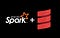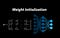# A Deep Dive Into Spark Datasets and DataFrames Using Scala

## A comprehensive guide to Spark datasets and DataFramesImage by Author

# Preliminary

Scala is a powerful programming language that combines functional and object-oriented programming. It is a JVM-based statistically typed language. Apache Spark is written in Scala, and because of its scalability on JVM, it is a popular programming language for data developers working on Spark projects. …

# Understanding Principal Component Analysis and its Application in Data Science — Part 2

## Learn the mathematical intuition behind PCA

In the first part of this article, I introduced the covariance matrix and discussed the mathematical foundation of PCA. In this part first, we discuss the application of singular value decomposition (SVD) in SVD and then we see a case study of PCA for the MNIST dataset. Finally, we discuss the multivariate normal distribution and the limitations of PCA. If I refer to a figure or equation or listing which cannot be found here, then you should look for that in the first part of the article. The Eqs. …

# Understanding Principal Component Analysis and its Application in Data Science — Part 1

## Learn the mathematical intuition behind PCA

Principal Component Analysis (PCA), is a method used to reduce the dimensionality of large datasets. We will also study the covariance matrix and the multivariate normal distribution in detail since understanding them will result in a better understanding of PCA. The Python scripts in this article show you how PCA can be implemented from scratch and using the Scikit Learn library.

Notation

Currently Medium supports subscripts and superscripts only for some characters. So to write the name of the variables, I use this notation: Every character after ^ is a superscript character and every character after _ (and before ^…

# Weight Initialization in Deep Neural Networks

## Learn about different weight initialization methods in neural networksImage by Author

Weight and bias are the adjustable parameters of a neural network, and during the training phase, they are changed using the gradient descent algorithm to minimize the cost function of the network. However, they must be initialized before one can start training the network, and this initialization step has an important effect on the network training. In this article, I will first explain the importance of the wight initialization and then discuss the different methods that can be used for this purpose.

Notation

Currently Medium supports superscripts only for numbers, and it has no support for subscripts. So to write…

# An Introduction to Deep Feedforward Neural Networks

## The mathematical foundation of feedforward neural nets

The feedforward neural network is the simplest type of artificial neural network which has lots of applications in machine learning. It was the first type of neural network ever created, and a firm understanding of this network can help you understand the more complicated architectures like convolutional or recurrent neural nets. This article is inspired by the Deep Learning Specialization course of Andrew Ng in Coursera, and I have used a similar notation to describe the neural net architecture and the related mathematical equations. This course is a very good online resource to start learning about neural nets, but since…

# Python Stack Frames and Tail-Call Optimization

## Avoiding stack overflow in Python using tail-recursion

Recursion in computer science is a method of problem-solving in which a function calls itself from within its own code. This method is very useful and can be applied to many types of problems, however, it has a limitation. Functions use the stack to keep their local variables, and the stack has a limited size. So if the recursion is too deep you will eventually run out of stack space which is called a stack overflow. However, some compilers implement tail-call optimization, allowing unlimited recursion to occur without stack overflow. …

# Understanding Python Bytecode

## Learn about disassembling Python bytecode

The source code of a programming language can be executed using an interpreter or a compiler. In a compiled language, a compiler will translate the source code directly into binary machine code. This machine code is specific to that target machine since each machine can have a different operating system and hardware. After compilation, the target machine will directly run the machine code.

In an interpreted language, the source code is not directly run by the target machine. There is another program called the interpreter that reads and executes the source code directly. …

# Closures and Decorators in Python

## You will finally understand what closures and decorators are

Closures are an important tool in functional programming, and some important concepts like currying and partial application can be implemented using them. Decorators are also a powerful tool in Python which are implemented using closures and allow the programmers to modify the behavior of a function without permanently modifying it. In this article, I will first explain the closures and some of their applications and then introduce the decorators.

Scope of variables

To better understand the closures first we need to learn about the scope of variables in Python. The scope of a variable refers to the area in which…

# Understanding Singular Value Decomposition and its Application in Data Science

## Learn about the intuition behind Singular Value Decomposition

In linear algebra, the Singular Value Decomposition (SVD) of a matrix is a factorization of that matrix into three matrices. It has some interesting algebraic properties and conveys important geometrical and theoretical insights about linear transformations. It also has some important applications in data science. In this article, I will try to explain the mathematical intuition behind SVD and its geometrical meaning. Instead of manual calculations, I will use the Python libraries to do the calculations and later give you some examples of using SVD in data science applications. In this article, bold-face lower-case letters (like a) refer to vectors…

# ROC Curve, a Complete Introduction

Written by Reza Bagheri

An ROC (Receiver Operating Characteristic) curve is a useful graphical tool to evaluate the performance of a binary classifier as its discrimination threshold is varied. To understand the ROC curve, we should first get familiar with a binary classifier and the confusion matrix. In binary classification, a collection of objects is given, and the task is to classify the objects into two groups based on their features. …## Reza Bagheri

Data Scientist and Researcher. LinkedIn: https://www.linkedin.com/in/reza-bagheri-71882a76/